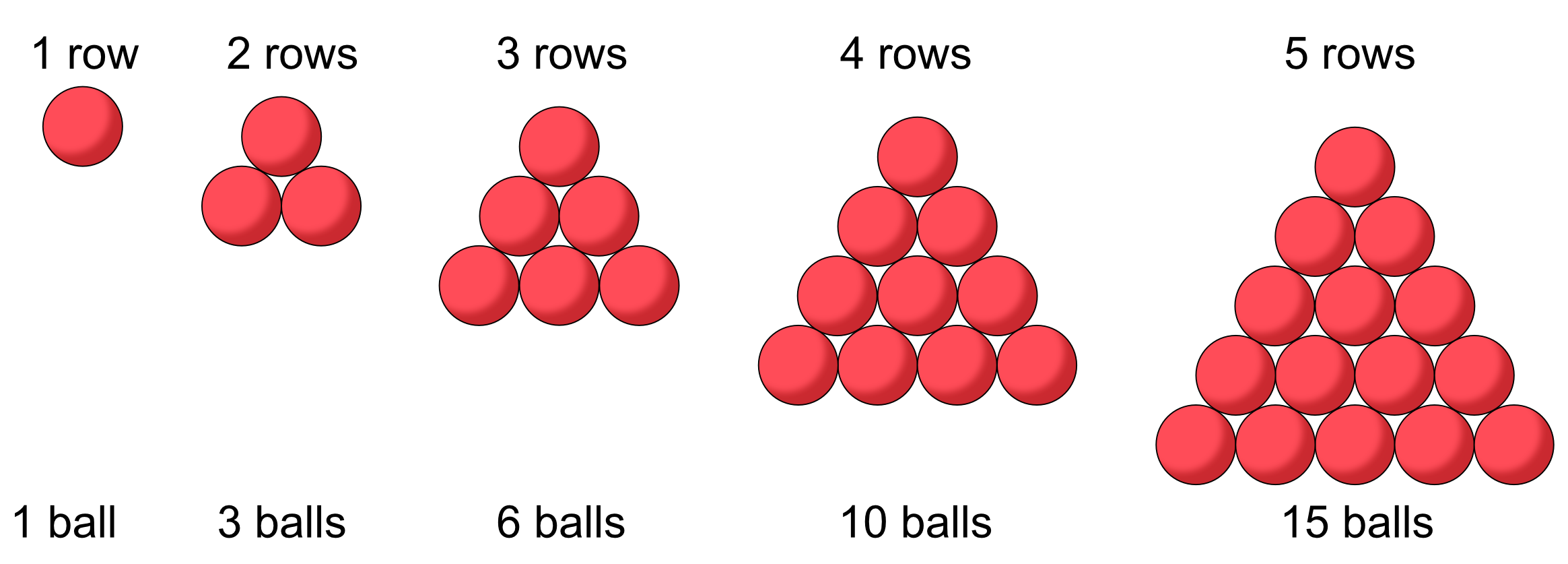Triangular Sequences

## Triangular Sequences

A triangular sequence is named after the shape made by individual balls packed together as close as possible.

For each new row, add one additional ball to the number of balls in the previous row.Any selected row equates to the term number. The value of the term is the total number of balls in the triangle up to and inlcuding that row.

## Example 1

What is the value of the 6th triangular number?

Add 6 to the fifth term, shown above. 15 + 6 = 21